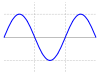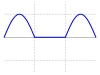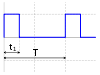# Crest factor

﻿
Crest factor

The crest factor or peak-to-average ratio (PAR) or peak-to-average power ratio (PAPR) is a measurement of a waveform, calculated from the peak amplitude of the waveform divided by the RMS value of the waveform.$C = {|x|_\mathrm{peak} \over x_\mathrm{rms}}$

It is therefore a dimensionless quantity. While this quotient is most simply expressed by a positive rational number, in commercial products it is also commonly stated as the ratio of two whole numbers, e.g., 2:1. In signal processing applications it is often expressed in decibels (dB).

The minimum possible crest factor is 1, 1:1 or 0 dB.

## Examples

This table provides values for some normalized waveforms:

Wave type Waveform Peak magnitude (normalized) RMS value Crest factor Crest factor (dB)
DC 1 1 1 0.0 dB
Sine wave1${1 \over \sqrt{2}} \approx 0.707$$\sqrt{2} \approx 1.414$ 3.01 dB
Full-wave rectified sine1${1 \over \sqrt{2}} \approx 0.707$$\sqrt{2} \approx 1.414$ 3.01 dB
Half-wave rectified sine1${1 \over 2} = 0.5$$2 \,$ 6.02 dB
Triangle wave1${1 \over \sqrt{3}} \approx 0.577$$\sqrt{3} \approx 1.732$ 4.77 dB
Square wave1 1 1 0 dB
PWM-Signal1$\sqrt{ \frac{t_1}T }$$\sqrt{ \frac T{t_1}}$$20\log\sqrt{ \frac T{t_1}}$ dB

QPSK 1 1 1 0 dB[citation needed]
8VSB 6.5–8.1 dB 
64QAM 1$\sqrt{ \frac{3}{7} }$$\sqrt{ \frac{7}{3} }$ 3.7 dB[citation needed]$\infty$-QAM 1$\sqrt{ \frac{1}{3} }$$\sqrt{ 3 }$ 4.8 dB[citation needed]
OFDM ~12 dB
GMSK 1 1 1 0 dB

Notes: 1. crest factors specified for QPSK, QAM, WCDMA are typical factors needed for reliable communication, not the theoretical crest factors which can be larger.

## Digital multimeters

Crest factor is an important parameter to understand when trying to take accurate measurements of low frequency signals. For example, given a certain digital multimeter with an AC accuracy of 0.03 % (always specified for sine waves) with an additional error of 0.2 % for crest factors between 1.414 and 5, then the total error for measuring a triangular wave (crest factor = 1.73) is 0.03 % + 0.2 % = 0.23 %. In acoustics, crest factor is usually expressed in decibels. For example, for a sine wave the 1.414 ratio is 20 log(1.414) or 3 dB. Most ambient noise has a crest factor of around 10 dB while impulsive sounds such as gunshots can have crest factors of over 30 dB. (Note the waveform factor of the half wave sine wave rectified signal should be 2.22 not 1.11)

## Peak-to-average ratio (PAR) meter

A peak-to-average ratio meter (Par meter) is a device used to measure the ratio of the peak power level to the time-averaged power level in an electrical circuit. This quantity is known as the peak-to-average ratio (p/a r or PAR). Such meters are used as a quick means to identify degraded telephone channels.

Par meters are very sensitive to envelope delay distortion. They may also be used for idle channel noise, nonlinear distortion, and amplitude-distortion measurements.

The peak-to-average ratio can be determined for many signal parameters, such as voltage, current, power, frequency, and phase.

## Applications

• Electrical engineering — for describing the quality of an AC power waveform
• Vibration analysis — for estimating the amount of impact wear in a bearing 
• Radio and audio electronics — for estimating the headroom required in a signal chain 
• Music has a widely-varying crest factor. Typical values for a processed mix are around 4–8 (which corresponds to 12–18 dB of headroom, usually involving audio level compression), and 8–10 for an unprocessed recording (18–20 dB).
• Physiology — for analysing the sound of snoring 

Wikimedia Foundation. 2010.

### Look at other dictionaries:

• Crest — may refer to: Contents 1 In science 2 In popular culture 3 In commerce 4 Places 5 Acronyms …   Wikipedia

• Crest (toothpaste) — Crest Tube of Crest Type Toothpaste Owner Procter Gamble Country U.S. Introduced …   Wikipedia

• Factor de transcripción asociado con microftalmia — HUGO 7105 Símbolo MITF Símbolos alt. WS2A …   Wikipedia Español

• Neural crest — The formation of neural crest during the process of neurulation. Neural crest is first induced in the region of the neural plate border. After neural tube closure, neural crest delaminates from the region between the dorsal neural tube and… …   Wikipedia

• Dairy Crest — Group plc Type Public (LSE: DCG) Industry D …   Wikipedia

• Cranial neural crest — Latin crista neuralis cranialis Code TE E5.15.1.0.2.0.1 The cranial neural crest is a form of neural crest. The cranial neural crest arises in the anterior and populates the face and the pharyngeal arches giving rise to bones, cartilage, n …   Wikipedia

• Max Factor — This article is about the brand. For the man, see Max Factor, Sr.. Max Factor Company is a cosmetics company, founded during 1909 by Maksymilian Faktorowicz (1877–August 30, 1938), Max Factor, a Polish Jewish cosmetician. Max Factor Company was a …   Wikipedia

• Microphthalmia-associated transcription factor — Identifiers Symbols MITF; MI; WS2; WS2A; bHLHe32 External IDs …   Wikipedia

• brain-derived neurotrophic factor — (= BDNF; BDGF) Small basic protein purified from pig brain; a member of the family of neurotrophins that also includes nerve growth factor and neurotrophin 3. In contrast to NGF, BDNF is predominantly (though not exclusively) localized in the… …   Dictionary of molecular biology

• Pink noise — This article is about the signal in information theory. For the band, see PINKNOISE. Colors of noise White Pink Red (Brownian) Grey Pink noise or 1/ƒ noise (sometimes also called flicker noise) is a signal or process with a frequency spectrum… …   Wikipedia